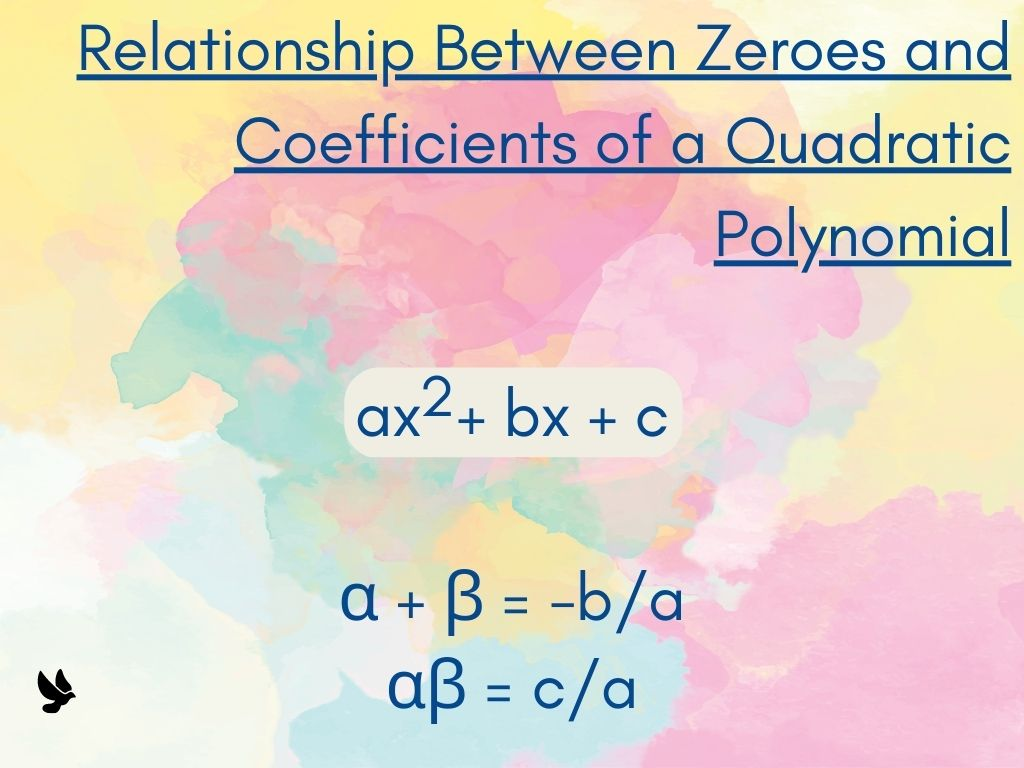## Relationship Between Zeroes and Coefficients of a Quadratic Polynomial Class 10th

Introduction We use the standard form of a quadratic polynomial to find the Relationship between Zeroes and Coefficients of a Quadratic Polynomial. We know the standard form of a quadratic polynomial is f(x) = ax2 + bx + c. Derivation of Formula Let α and β be the two zeroes of this polynomial. Then (x – …